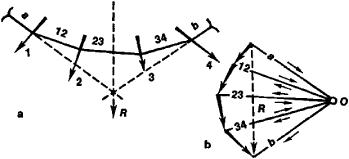# Funicular Polygon

Also found in: Dictionary.

## funicular polygon

[fə′nik·yə·lər ′päl·ə‚gän]
(mechanics)
The figure formed by a light string hung between two points from which weights are suspended at various points.
A force diagram for such a string, in which the forces (weights and tensions) acting on points of the string from which weights are suspended are represented by a series of adjacent triangles.
McGraw-Hill Dictionary of Scientific & Technical Terms, 6E, Copyright © 2003 by The McGraw-Hill Companies, Inc.
The following article is from The Great Soviet Encyclopedia (1979). It might be outdated or ideologically biased.

## Funicular Polygon

a graphical method of finding the reactive forces and resultants of a system of forces, constructing bending-moment diagrams, determining the rational shapes of arched and suspended systems, and solving other problems of the statics of two-dimensional systems.

The construction of funicular polygons is based on the conception of a polygon formed by an axis that is fixed at the ends of a weightless thread (string), which is tensioned by the forces acting on it. The construction of a funicular polygon together with a force polygon (see Figure 1) is also used to determine the geometric characteristics of planar cross sections and to solve certain problems in engineering hydraulics, economics, and other fields.Figure 1. (a) Funicular polygon; (b) force polygon